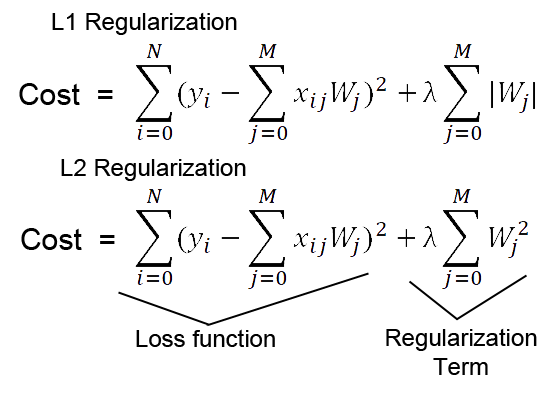# L1 vs L2 Regularization: Which is better

Original article can be found here (source): Deep Learning on Medium# L1 vs L2 Regularization: Which is better

A lot of people usually get confused which regularization technique is better to avoid overfitting while training a machine learning model.

I won’t go about much in detail about the maths side. Instead will try to explain the intuitive difference between them.

The main intuitive difference between the L1 and L2 regularization is that L1 regularization tries to estimate the median of the data while the L2 regularization tries to estimate the mean of the data to avoid overfitting.

As we can see from the formula of L1 and L2 regularization, L1 regularization adds the penalty term in cost function by adding the absolute value of weight(Wj) parameters, while L2 regularization adds the squared value of weights(Wj) in the cost function.

While taking derivative of the cost function, in L1 regularization it will estimate around the median of the data. Let me explain it in this way — Suppose you take an arbitrary value from the data (assume data is spread along a horizontal line). If you then move in one direction to some distance d, suppose in the backward direction, then while calculating loss, the values to the one side (let say left side) of the chosen point will have a lesser loss value while on another side will contribute more in the loss function calculation.

Therefore, to minimize the loss function, we should try to estimate a value that should lie at the mid of the data distribution. That value will also be the median of the data distribution mathematically.

While in L2 regularization, while calculating the loss function in the gradient calculation step, the loss function tries to minimize the loss by subtracting it from the average of the data distribution.

That’s the main intuitive difference between the L1 (Lasso) and L2 (Ridge) regularization technique.

Another difference between them is that L1 regularization helps in feature selection by eliminating the features that are not important. This is helpful when the number of feature points are large in number.

That’s all from my side this time. Hope I am able to explain the intuitive difference between both the regularization techniques.